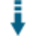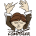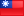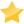# PHP Dependency Injection Calculator: Perform math calculations using an injected objectDownload .zip
 InfoDocumentationView files (9)Download .zip Reputation Support forum Blog (1) Links
 Last Updated Ratings Unique User Downloads Download Rankings 2023-05-31 (3 months ago)Not yet rated by the users Total: 34 All time: 10,812 This week: 94Version License PHP version Categories dependency-calculato 1.0.0 MIT/X Consortium ... 7 Math, Design Patterns, PHP 7
Description

Author

peter279k

Contributor

This package can perform math calculations using an injected object.

It provides an abstract calculator class that can perform several math calculations with numbers, like adding, subtracting, multiplying, and dividing.

The calculator class takes an injected object that will perform the actual calculations.

The package provides several classes that implement the math operations using different approaches to perform those operations.

Currently, it provides math operations classes that can be used to create objects to inject in the calculator class, like:

- GMP (GNU Multi Precision)

- BCMath (Arbitrary Precision Mathematics)

Innovation AwardJune 2023 Number 6 Several solutions can work as alternatives when a developer needs to solve a problem. For instance, a developer may store and retrieve the application data using different databases. In this case, using different classes that implement the same database access operations with other codes is recommended for accessing different types of databases. Then the application may write code that works well with different databases. If, in the future, the developer needs to change the application to use a different type of database, he needs to use an object to access that type of database without needing to change the code of the rest of the application. In this case, the application uses a database access object and injects it into the code that implements the application. This approach to separate the application code from the implementation class code that the application depends on is called dependency injection. This package implements this approach to perform math operations. It provides a main class that implements several types of math operations. It also offers several courses that implement those math operations with different PHP extensions that provide various types of precision. Manuel LemosPerformance Level
Name: Classes: Chun-Sheng, Li `` 22 packages by Chun-Sheng, LiTaiwan 30 2413 6 in Taiwan682 in TaiwanLevel 1Innovation awardNominee: 12x

Winner: 1x

Details

# Dependency Calculator

## Usage

Here is some examples about how to calculate two numbers.

``````use Lee\Calculator\Calculator;
use Lee\Calculator\modules\GmpCalculator;
use Lee\Calculator\modules\BcMathCalculator;

\$gmpCalculator = new Calculator(new GmpCalculator());
\$bcMathCalculator = new Calculator(new BcMathCalculator());

``````

### Minus two numbers

``````use Lee\Calculator\Calculator;
use Lee\Calculator\modules\GmpCalculator;
use Lee\Calculator\modules\BcMathCalculator;

\$gmpCalculator = new Calculator(new GmpCalculator());
\$bcMathCalculator = new Calculator(new BcMathCalculator());

echo \$gmpCalculator->minus('123456789', '123456789000000'); // -123456665543211
echo \$bcMathCalculator->minus('123456789', '123456789000000'); // -123456665543211
``````

### Multiple two numbers

``````
use Lee\Calculator\Calculator;
use Lee\Calculator\modules\GmpCalculator;
use Lee\Calculator\modules\BcMathCalculator;

\$gmpCalculator = new Calculator(new GmpCalculator());
\$bcMathCalculator = new Calculator(new BcMathCalculator());

echo \$gmpCalculator->mul('123456789', '123456789000000'); // 15241578750190521000000
echo \$bcMathCalculator->mul('123456789', '123456789000000'); // 15241578750190521000000
``````

### Divide two numbers

``````
use Lee\Calculator\Calculator;
use Lee\Calculator\modules\GmpCalculator;
use Lee\Calculator\modules\BcMathCalculator;

\$gmpCalculator = new Calculator(new GmpCalculator());
\$bcMathCalculator = new Calculator(new BcMathCalculator());

echo \$gmpCalculator->divide('123456789000000', '123456789'); // 1000000
echo \$bcMathCalculator->divide('123456789000000', '123456789'); // 1000000
``````Files
File Role Description.github (1 directory)src (1 file, 2 directories)tests (1 file)composer.json Data Auxiliary dataphpunit.xml.dist Data Auxiliary dataREADME.md Doc. DocumentationFiles / .github
File Role Descriptionworkflows (1 file)Files / .github / workflows
File Role Descriptionbuild.yml Data Auxiliary dataFiles / src
File Role Descriptioninterfaces (1 file)modules (2 files)Calculator.php Class Class sourceFiles / src / interfaces
File Role DescriptionCalculator.php Class Class sourceFiles / src / modules
File Role DescriptionBcMathCalculator.php Class Class sourceGmpCalculator.php Class Class sourceFiles / tests
File Role DescriptionCalculatorTest.php Class Class source

 All time: 10,812 This week: 94About usAdvertise on this siteSite mapNewsletterStatisticsSite tipsPrivacy policyContact
For more information send a message to `info at phpclasses dot org`.